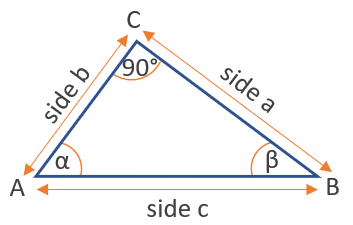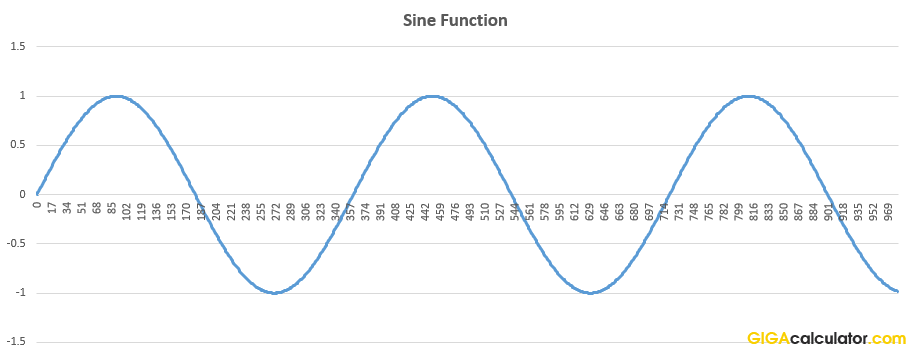# Trigonometry Calculator

Use this trigonometry calculator to easily calculate trigonometry functions in degrees or radians.

### Calculation results

1 sin(90) =
Share calculator:

Embed this tool:
get code

## Trigonometry functions

The main trigonometric functions are sine, cosine, and tangent, often written as sin(x), cos(x), and tan(x). The common thing for them is that they express the ratios between different sides of a right-angled triangle, from the point of view of the angle of interest, and thus some of them are transformations of the others. For example tan(x) = sin(x) / cos(x). Our trigonometric calculator supports all three major functions.

These functions have a lot of practical applications in geometry, physics, and computer science. The sine function is used to model sound waves, earthquake waves, and even temperature variations. The cosine has uses in audio, video, and image compression algorithms such as those used in JPEG, MP3, WMV, MPEG, and others. The tangent is used in measuring distances and paths, including in aviation.In the figure above, sin(α) = a/c, cos(α) = b/c, while tan(α) = a/b.

#### Sine wave graphAbove is the famous sine wave, while below you can see the more unfamiliar tangent function graph, which looks like a stretched sideways "s".

## Reciprocal trigonometric functions

The reciprocal functions of the sine, cosine, and tangent are, respectively, the cosecant, secant, and the cotangent. They are of limited practical use nowadays, given our calculation capabilities, but are still a part of the school curriculum in high schools and universities. Our calculator supports them for your convenience.

## Inverse trigonometric functions

The inverse trigonometric functions, sometimes referred to as antitrigonometric or arcus functions, include arcsine, arcosine, arctangent, and arccotangent, usually written as arcsin(x), arccos(x), arctan(x) and arccot(x). They have a more limited usage since the invention of the calculator and computers, which make the calculation of the three main trigonometric functions trivial.

#### Cite this calculator & page

If you'd like to cite this online calculator resource and information as provided on the page, you can use the following citation:
Georgiev G.Z., "Trigonometry Calculator", [online] Available at: https://www.gigacalculator.com/calculators/trigonometry-calculator.php URL [Accessed Date: 06 Jun, 2023].